# Introduction

A seminal paper by John Rick some 30 years ago (1987) first introduced the idea of using the frequency of archaeological radiocarbon dates through time as a proxy for highs and lows in human population. The increased availability of large collections of archaeological (especially anthropogenic) radiocarbon dates has dramatically pushed this research agenda forward in recent years. New case studies from across the globe are regularly being published, stimulating the development of new techniques to tackle specific methodological and interpretative issues.

rcarbon is an R package for the analysis of large collections of radiocarbon dates, with particular emphasis on this “date as data” approach. It offers basic calibration functions as well as a suite of statistical tests for examining aggregated calibrated dates, using the method commonly referred to as summed probability distributions of radiocarbon dates (SPDs).

Stable versions of the rcarbon package can be directly installed from CRAN (using the command install.packages("rcarbon")), whilst the development version can be installed from the github repository using the following command (the function requires the devtools package):

devtools::install_github("ahb108/rcarbon")

Note that the development version can be unstable, so for general use we recommend the CRAN version.

Once the installation is completed the package can be loaded using the library() command:

library(rcarbon)

# Calibrating $$^{14}$$C Dates

Single or multiple radiocarbon dates can be calibrated using the calibrate() function, which uses the probability density approach (see Bronk Ramsey 2008) implemented in most calibration software (e.g. OxCal) as well as in other R packages (especially Bchron. Note that the latter R package also provides age-depth modelling for environmental cores and experimental options for aggregating dates via Gaussian mixtures.

The example below calibrates a sample with a $$^{14}$$C Age of 4200 BP and an error of 30 years using the intcal13 calibration curve:

x <- calibrate(x=4200,errors=30,calCurves='intcal13')

The resulting object of class CalDates can then be plotted using the basic plot() function (in this case highlighting the 95% higher posterior density interval):

plot(x,HPD=TRUE,credMass=0.95)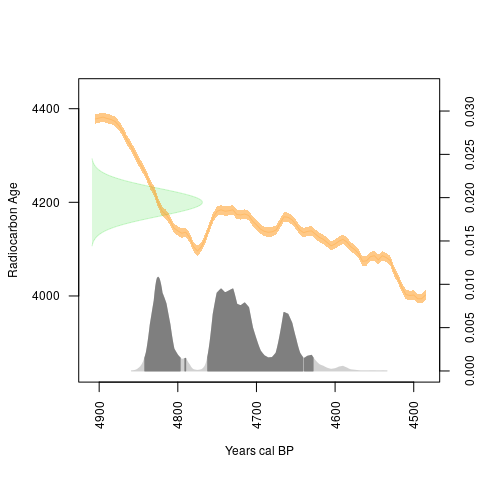Multiple dates can be calibrated by supplying a vector of numerical values, and the summary() function can be used to retrieve one and the two sigma ranges as well as the median calibrated date:

xx <- calibrate(x=c(5700,4820,6450,7200),errors=c(30,40,40,30),calCurves='intcal13')
summary(xx)
##   DateID MedianBP OneSigma_BP_1 OneSigma_BP_2 TwoSigma_BP_1 TwoSigma_BP_2
## 1      1     6478  6528 to 6521  6508 to 6437  6596 to 6595  6564 to 6407
## 2      2     5527  5599 to 5580  5528 to 5483  5642 to 5628  5615 to 5566
## 3      3     7367  7422 to 7411  7397 to 7328  7430 to 7287      NA to NA
## 4      4     8002  8020 to 7973      NA to NA  8150 to 8143  8128 to 8122
##   TwoSigma_BP_3 TwoSigma_BP_4
## 1      NA to NA      NA to NA
## 2  5562 to 5470      NA to NA
## 3      NA to NA      NA to NA
## 4  8108 to 8093  8057 to 7952

The calibrate() function can also be executed in parallel by specifying the number of cores using the argument ncores.

Individual calibrated dates stored in the CalDates class objects can be extracted using square brackets (e.g. xx to extract the second date). The package also provides a subset() method and a which.CalDates() function to subset or identify specific dates with a given probability mass within a given interval. The example below extracts all dates from the object xx with a cumulative probability mass of 0.5 or over between 7000 and 5500 cal BP:

which.CalDates(xx,BP<=7000&BP>=5500,p=0.5)
##  1 2
xx2 = subset(xx,BP<=7000&BP>=5500,p=0.5)
summary(xx2)
##   DateID MedianBP OneSigma_BP_1 OneSigma_BP_2 TwoSigma_BP_1 TwoSigma_BP_2
## 1      1     6478  6528 to 6521  6508 to 6437  6596 to 6595  6564 to 6407
## 2      2     5527  5599 to 5580  5528 to 5483  5642 to 5628  5615 to 5566
##   TwoSigma_BP_3
## 1      NA to NA
## 2  5562 to 5470

Calibration can be done using different calibration curves. The following example is for a marine sample with $$\Delta R = 340\pm20$$:

x <- calibrate(4000,30,calCurves='marine13',resOffsets=340,resErrors=20)
plot(x,HPD=TRUE,calendar="BCAD") #using BC/AD instead of BP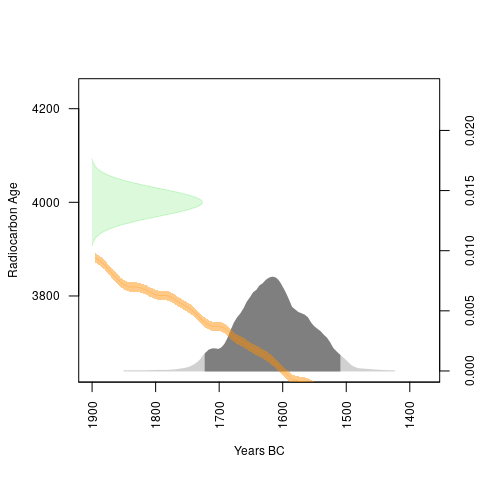Users can also supply their own custom calibration curves. The example below uses a mixed marine/terrestrial curve generated using the mixCurves() function:

#generate 70% terrestrial and 30% marine curve
myCurve <- mixCurves('intcal13','marine13',p=0.7,resOffsets=340,resErrors=20)
plot(calibrate(4000,30,calCurves=myCurve))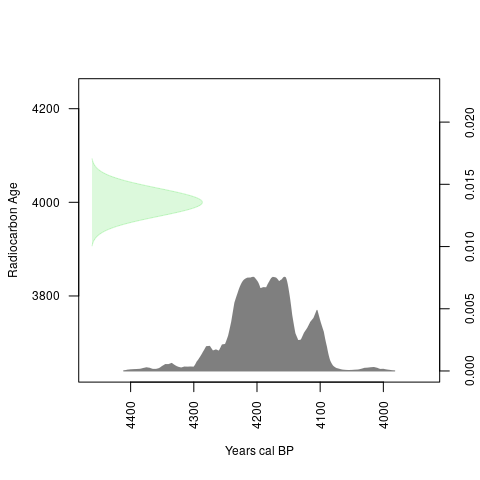### Normalisation

By default, calibrated probabilities are normalised so the total probability is equal to one, in step with most other radiocarbon calibration software. However, Weninger et al (2015) argue that when dates are aggregated by summation, this normalisation process can generate artificial spikes in the resulting summed probability distributions (SPDs) coinciding with steeper portions of the calibration curve. By specifying normalised=FALSE in calibrate() it is possible to obtain unnormalised calibrations. Using normalised or unnormalised calibrations does not have an impact on the shape of each individual dates calibrated probability distribution, but does influence the shape of SPDs, so we suggest at minimum that any case study should explore whether its results differ much when normalised versus unnormalised dates are used.

# Aggregating $$^{14}$$C Dates: Summed Probability Distributions (SPD)

The function spd() aggregates (sums) calibrated radiocarbon dates within a defined chronological range. The resulting object can then be displayed using the plot() function. The example below uses data from the EUREOVOL project database (Manning et al 2016) which can be directly accessed within the package.

data(euroevol)
DK=subset(euroevol,Country=="Denmark") #subset of Danish dates
DK.caldates=calibrate(x=DK$C14Age,errors=DK$C14SD,calCurves='intcal13',ncores=3) #running calibration over 3 cores
DK.spd = spd(DK.caldates,timeRange=c(8000,4000))
plot(DK.spd)
plot(DK.spd,runm=200,add=TRUE,type="simple",col="darkorange",lwd=2,lty=2) #using a rolling average of 200 years for smoothing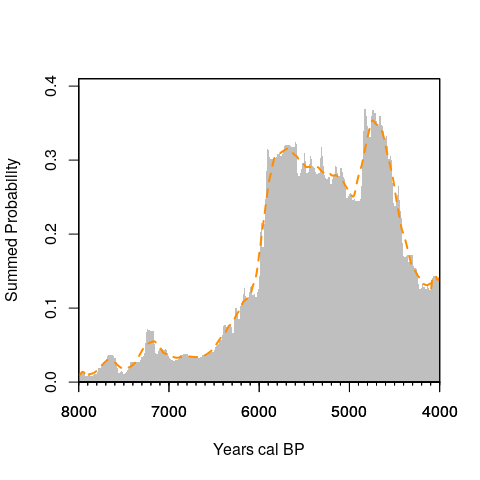It is also possible to limit the plot of the SPD to a particular window of time and/or use a 'BC/AD' timescale:

# show SPD between 6000 and 3000 BC
plot(DK.spd,calendar='BCAD',xlim=c(-6000,-3000))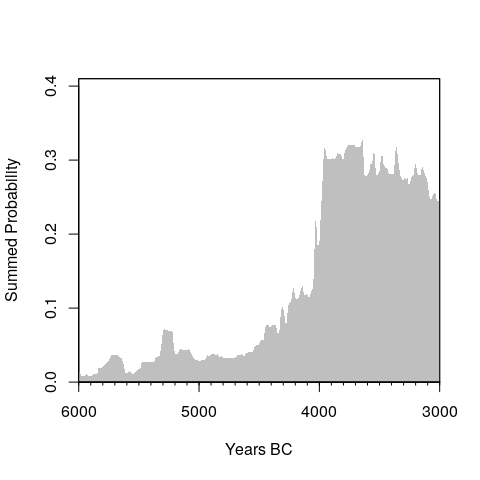# show SPD between 7000 and 5000 BP
# plot(DK.spd,calendar='BP',xlim=c(7000,5000)) 

## Binning

SPDs can be potentially biased if there is strong inter-site variability in sample size, for example where one well-resourced research project has sampled one particular site for an unusual number of dates. This might generate misleading peaks in the SPD and to mitigate this effect it is possible to create artificial bins, a local SPD based on samples associated with a particular site and close in time that is divided by the number of dates in the bin or to the average SPD (in case of non-normalised calibration). Dates are assigned to the same or different bins based on their proximity to one another in (either $$^{14}$$C in time or median calibrated date) using hierarchical clustering with a user-defined cut-off value (using the hclust() function and the argument h) and this binning is implemented by the binPrep() function. The code below illustrates an example using a cut-off value of 100 years:

DK.bins = binPrep(sites=DK$SiteID,ages=DK$C14Age,h=100)
# DK.bins = binPrep(sites=DK$SiteID,ages=DK.caldates,h=100) #using median calibrated date The resulting object can then be used as an argument for the spd() function: DK.spd.bins = spd(DK.caldates,bins=DK.bins,timeRange=c(8000,4000)) plot(DK.spd.bins)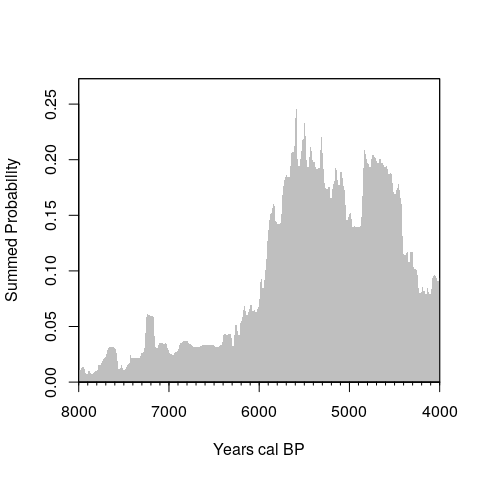The selection of appropriate cut-off values for binning has not been discussed in the literature (Shennan et al 2013 uses a value of 200 years but their method is slightly different). From a practical point of view, a “bin” represents a “phase” or episode of occupation (at an archaeological site, for example), but clearly, this is a problematic definition in the case of continuous occupation. The binning process should hence be used with caution, and its implications should be explored via a sensitivity analysis. The function binsense() enables a visual assessment of how different cut-off values can modify the shape of the SPD. The example below explores six different values and show how the highest peak in the SPD changes as a function of h but the overall dynamics remains essentially the same. binsense(x=DK.caldates,y=DK$SiteID,h=seq(0,500,100),timeRange=c(8000,4000))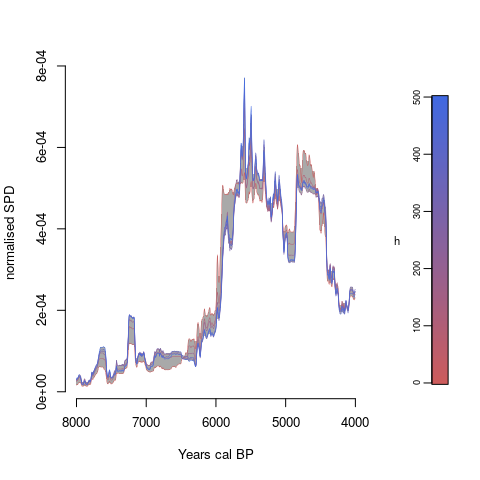### Visualising Bins

The location (in time) of individual bins can be shown by using the binMed() and the barCodes() functions. The former computes the median date from each bin while the latter display them as vertical lines on an existing SPD plot (inspired by a method first used in the CalPal radiocarbon software package).

Dk.bins.med=binMed(x = DK.caldates,bins=DK.bins)
plot(DK.spd.bins,runm=200)
barCodes(Dk.bins.med,yrng = c(0,0.01))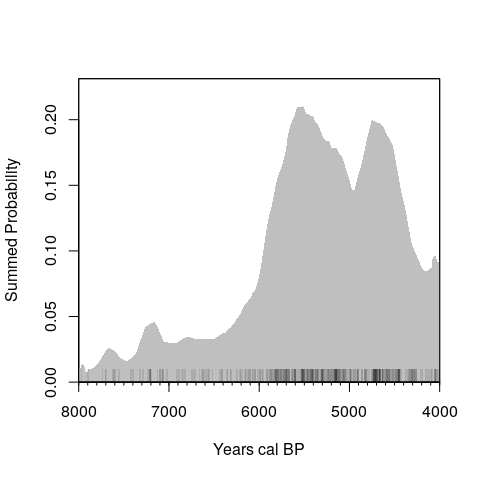### Thinning

An alternative way to tackle inter-site variation in sampling intensity is to set a maximum sample size per site or bin. This can be achieved by using the thinDates() function which returns index values based on a variety of criteria. The example below randomly selects one date from each bin and constructs an SPD without binning:

# subset CalDates object based on random thinning
DK.caldates2 = DK.caldates[thinDates(ages=DK$C14Age, errors=DK$C14SD, bins=DK.bins, size=1, method='random')]
# aggregation and visualisation of the SPD
DK.spd.thinned = spd(DK.caldates2,timeRange=c(8000,4000))
##  "Extracting and aggregating..."
##  "Done."
plot(DK.spd.thinned)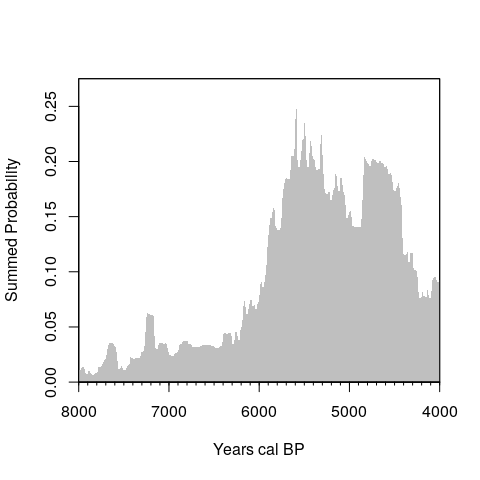## Composite Kernel Density Estimates (CKDE)

An alternative approach to visualising the changing frequencies of radiocarbon dates is to create a composite kernel density estimate (CKDE, Brown 2017). The methods consist of randomly sampling calendar dates from each calibrated date and generate a kernel density estimate (KDE) with a user-defined bandwidth. The process is iterated multiple times and the resulting set of KDEs is visualised as an envelope. To generate a CKDE using the rcarbon package we first use the sampleDates() function. This generates multiple sets of random dates that can then be used to generate the CKDE. If the bin argument is supplied the function generates random dates from each bin rather than each date:

DK.randates = sampleDates(DK.caldates,bins=DK.bins,nsim=100,verbose=FALSE)

The resulting object becomes the key argument for the ckde() function, whose output can be displayed via a generic plot() function:

D.ckde = ckde(DK.randates,timeRange=c(8000,4000),bw=200)
plot(D.ckde,type='multiline')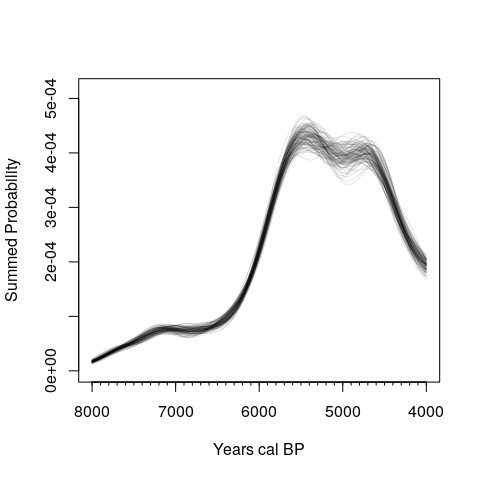The rcarbon package also enables bootstrapping (by settingboot=TRUE in sampleDates()), and the CKDE can be weighted (by settingnormalised=FALSE in ckde(); although the calibrated dates supplied to sampleDates() should also not be normalised) to emulate an SPD with non-normalised dates. For further details, please read the help documentation of the two functions.

# Hypothesis Testing

The shape of empirical SPDs can be affected by a host of possible biases including taphonomic loss, sampling error, and the shape of the calibration curve. One way to approach this problem is to assess SPDs in relation to theoretical expectations and adopt a hypothesis-testing framework. rcarbon provides several functions for doing this.

## Testing against theoretical growth models

Shennan et al 2013 (Timpson et al 2014 for more detail and methodological refinement) introduced a Monte-Carlo simulation approach consisting of a three-stage process: 1) fit a growth model to the observed SPD, for example via regression; 2) generate random samples from the fitted model; and 3) uncalibrate the samples. The resulting set of radiocarbon dates can then be calibrated and aggregated in order to generate an expected SPD of the fitted model that takes into account idiosyncrasies of the calibration process. This process can be repeated $$n$$ times to generate a distribution of SPDs (which takes into account the effect of sampling error) that can be compared to the observed data. Higher or lower than expected density of observed SPDs for a particular year will indicate local divergence of the observed SPD from the fitted model, and the magnitude and frequency of these deviations can be used to assess the goodness-of-fit via a global test. rcarbon implements this routine with the function modelTest(), which enables testing against exponential, linear, uniform, and user-defined custom models. The script below shows an example with the Danish SPD fitted to an exponential growth model:

nsim = 100
expnull <- modelTest(DK.caldates, errors=DK$C14SD, bins=DK.bins, nsim=nsim, timeRange=c(8000,4000), model="exponential",runm=100) ## Warning in modelTest(DK.caldates, errors = DK$C14SD, bins = DK.bins, nsim =
## nsim, : edgeSize reduced

We can extract the global p-value from the resulting object which can also be plotted.

plot(expnull)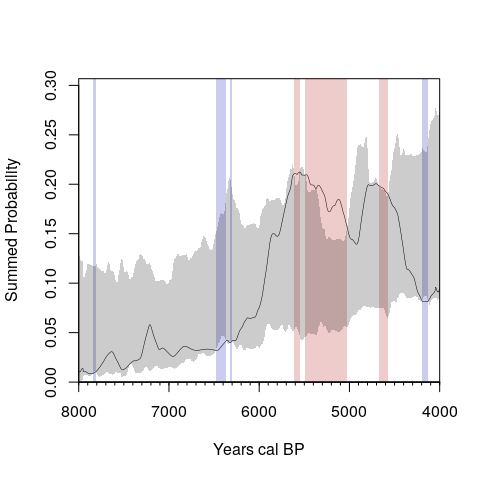expnull$pval #global p-value ##  0.02970297 The grey shaded region depicts the critical envelope that encompasses the middle 95% of the simulated SPDs, with red and blue regions highlighting portions of the SPD where positive and negative deviations are detected. Further details can be extracted using the summary() function: summary(expnull) ## 'modelTest()' function summary: ## ## Number of radiocarbon dates: 699 ## Number of bins: 443 ## ## Statistical Significance computed using 100 simulations. ## Global p-value: 0.0297. ## ## Signficant positive local deviations at: ## 5611~5553 BP ## 5490~5373 BP ## 5370~5026 BP ## 4676~4572 BP ## ## Significant negative local deviations at: ## 7840~7812 BP ## 6481~6365 BP ## 6327~6305 BP ## 4201~4130 BP ### Testing against custom growth models The modelTest() function can also be used to test user-defined theoretical growth models. The example below fits a logistic growth model (using the nls() function). # Generate a smoothed SPD DK.spd.smoothed = spd(DK.caldates,timeRange=c(8000,4000),bins=DK.bins,runm=100) # Start values should be adjusted depending on the observed SPD logFit <- nls(PrDens~SSlogis(calBP, Asym, xmid, scale),data=DK.spd.smoothed$grid,control=nls.control(maxiter=200),start=list(Asym=0.2,xmid=5500,scale=-100))
# Generate a data frame containing the fitted values
logFitDens=data.frame(calBP=DK.spd.smoothed$grid$calBP,PrDens=SSlogis(input=DK.spd.smoothed$grid$calBP,Asym=coefficients(logFit),xmid=coefficients(logFit),scal=coefficients(logFit)))
# Use the modelTest function (returning the raw simulation output - see below)
LogNull <- modelTest(DK.caldates, errors=DK$C14SD, bins=DK.bins,nsim=nsim, timeRange=c(8000,4000), model="custom",predgrid=logFitDens, runm=100, raw=TRUE) # Plot results plot(LogNull)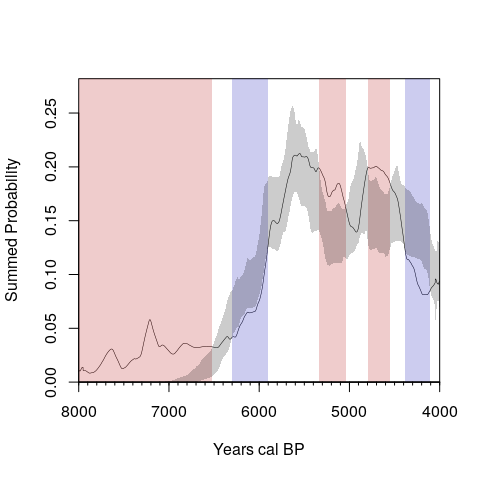# Retrieve p-values LogNull$pval
##  0.00990099

### Testing Local Growth Rates

The modelTest() function also enables the statistical comparison between the observed and expected growth rates of the fitted model. The rate is based on the comparison of the summed probability observed at each focal year $$t$$ against the summed probability at $$t-\Delta$$, where $$\Delta$$ is defined by the argument backsight in modelTest() a the range is calculated using the expression defined in the argument changexpr. The default values for backsight an changexpr are 50 years and the expression (t1/t0)^(1/d) - 1 where t1 is the summed probability for each year (i.e. $$t$$), t0 is the summed probability of the backsight year (i.e. $$t-\Delta$$), and d is the distance between the two time points (i.e. $$\Delta$$). The output of the growth rates analysis can be assessed by setting the argument type in the summary() and in the plot() functions. For example:

summary(expnull,type='roc')
## 'modelTest()' function summary:
##
## Number of radiocarbon dates: 699
## Number of bins: 443
## Backsight size: 50
##
## Statistical Significance computed using 100 simulations.
## Global p-value (rate of change): 0.9802.
##
## No significant positive local deviations
## No significant positive local deviations
plot(expnull,type='roc')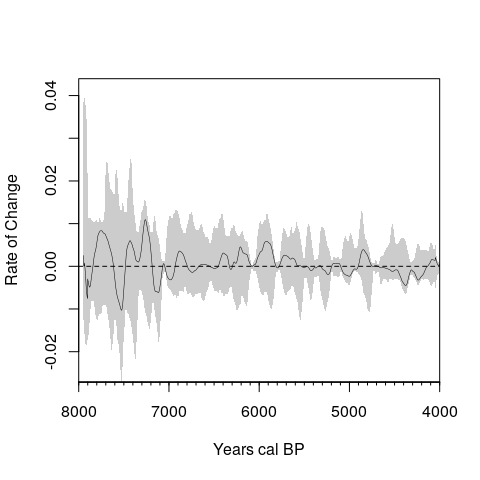### Point-to-Point Test

The modelTest() function does not itself indicate whether the observed difference between two particular points in time is significant, as both local and global tests are based on the overall shape of the observed and expected SPDs (or their corresponding rates of change). The p2pTest() follows a procedure introduced by Edinborough et al (2017) which compares the expected and the observed difference in radiocarbon density between just two user-defined points in time. The example below is based on the Danish subset using a uniform theoretical model.

#Fit a Uniform model (the argumet raw should be set to TRUE for p2pTest())
uninull <- modelTest(DK.caldates, errors=DK$C14SD, bins=DK.bins, nsim=nsim, timeRange=c(8000,4000), model="uniform",runm=100, raw=TRUE) ## Warning in modelTest(DK.caldates, errors = DK$C14SD, bins = DK.bins, nsim =
## nsim, : edgeSize reduced
#Test Difference between 5120 and 4920 cal BP
results=p2pTest(uninull,p1=5120,p2=4920)
results
## $p1 ##  5120 ## ##$p2
##  4920
##
## $pval ##  0.01980198 Note that when the arguments p1 and p2 are not supplied p2pTest() displays the SPD and enable users to select the two points on the plotted SPD interactively. ## Comparing empirical SPDs against each other SPDs are often compared against each other to evaluate regional variations in population trends (e.g.Timpson et al 2015) or to determine whether the relative proportion of different dated materials changes across time. Collard et al (2010) for instance demonstrates that the relative frequencies of different kinds of archaeological site have varied over time in Britain, whilst Stevens and Fuller (2012) argue that the proportion of wild versus domesticated crops fluctuated during the Neolithic (see also Bevan et al. 2017). The permTest() function provides a permutation test for comparing two or more SPDs, returning both global and local p-values using similar procedures to modelTest() (first introduced by Crema et al. 2016). The example below reproduces the analyses of Eastern Mediterranean dates by Roberts et al (2018): data(emedyd) # load data cal.emedyd = calibrate(emedyd$CRA,emedyd$Error,ncores=3,normalised=FALSE) bins.emedyd = binPrep(ages = emedyd$CRA,sites = emedyd$SiteName,h=50) perm.emedyd=permTest(x=cal.emedyd,marks=emedyd$Region,timeRange=c(16000,9000),bins=bins.emedyd,nsim=nsim,runm=50)
summary(perm.emedyd)
par(mfrow=c(3,1))
plot(perm.emedyd,focalm = 1,main="Southern Levant")
plot(perm.emedyd,focalm = 2,main="Northern Levant/Upper Mesopotamia")
plot(perm.emedyd,focalm = 3,main="South-Central Anatolia")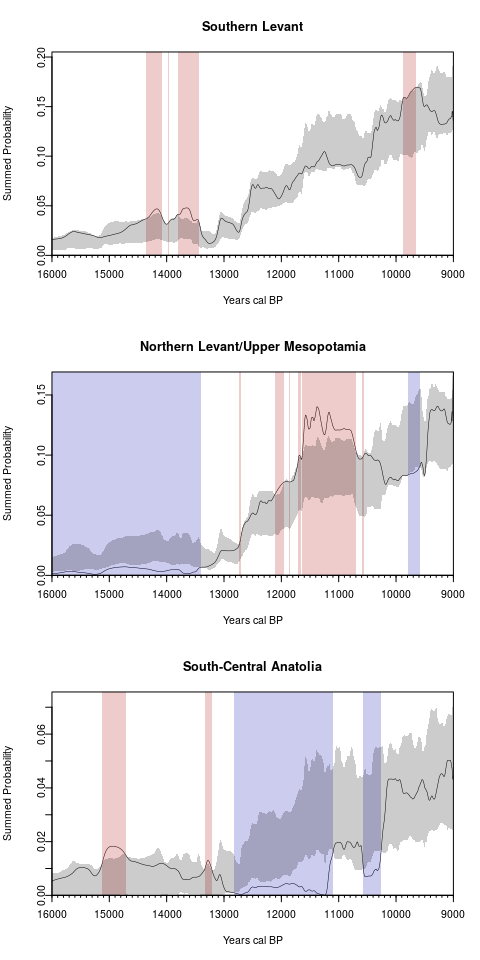As for modelTest(), it is also possible to compare the growth rates rather than the SPD:

par(mfrow=c(3,1))
plot(perm.emedyd,focalm = 1,main="Southern Levant", type='roc')
plot(perm.emedyd,focalm = 2,main="Northern Levant/Upper Mesopotamia", type='roc')
plot(perm.emedyd,focalm = 3,main="South-Central Anatolia", type='roc')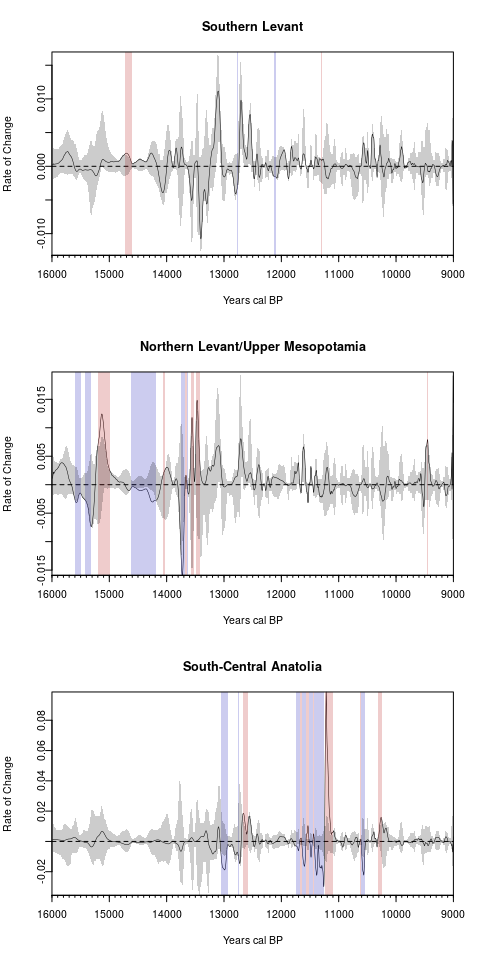## Spatial Analysis

The core principles of the "dates as data" approach can be extended over space by interpreting regions of high or low concentrations of dates across multiple temporal slices as evidence of higher or lower population densities. As in the case of ordinary SPDs, the visual inspection of such SPD maps can be problematic, as peaks and troughs in the density of radiocarbon dates can potentially be the result of calibration processes or sampling error. The problem is further exacerbated when the spatial window of analysis becomes larger, as differences in sampling design and intensity can hinder the observed pattern. The rcarbon package offers two techniques that take into account these issues when assessing spatio-temporal patterns in the density of radiocarbon dates.

### Spatio-Temporal Kernel Density Estimates

The stkde() function enables the computation of spatio-temporal kernel density estimates (KDE) of radiocarbon dates for a particular focal year based on user-defined spatial (sbw) and temporal (tbw) bandwidths. In practice, this is achieved by placing a Gaussian kernel around a chosen year and then the degree of overlap between this kernel and the probability distribution of each date is evaluated. The weights are then used to compute weighted spatial kernel density estimates using the density() function of the spatstat package (Baddeley et al 2015). The function returns the KDE for each focal year, the rate of change (based on the user-defined expression provided in the argument changepr) between focal years and some earlier backsight year, as well as relative risk surfaces (Kelsall and Diggle 1995). The latter consist of dividing the KDE of a particular year with the KDE of all periods to take into account overall differences in sampling intensity (see Chaput et al 2015 and Bevan et 2017 for examples).

The example below examines a subset of the EUROEVOL radiocarbon dates from England and Wales between 6500 to 5000 BP, using a temporal bandwidth of 50 years, a spatial bandwidth of 40km, and backsight of 200 years (i.e. change is computed between focal year and focal year minus 200 years):

## Load Data
data(ewdates)
data(ewowin)

## Calibrate and bin
x <- calibrate(x=ewdates$C14Age, errors=ewdates$C14SD, normalised=FALSE,verbose=FALSE)
bins1 <- binPrep(sites=ewdates$SiteID, ages=ewdates$C14Age, h=50)

## Create centennial timeslices (see help doc for further argument details)
stkde1 <- stkde(x=x, coords=ewdates[,c("Eastings", "Northings")], win=ewowin, sbw=40000, cellres=2000, focalyears=seq(6500, 5000, -100), tbw=50, bins=bins1, backsight=200, outdir=tempdir(), amount=1, verbose=FALSE)

The actual KDE time-slices are stored by the stkde() function in a user-defined directory defined by the argument outdir (the example above uses a temporary directory via the tempdir() function). The plot() function can then be used to display a variety of maps. The example below shows the focal intensity at year 5900 cal BP, the overall intensity across all years, the relative risk surface ( focal proportion ), and the rate of change between 5700 and 5900 cal BP ( focal change ).

par(mar=c(0.5, 0.5, 2.5, 2))
plot(stkde1, 5900, type="all")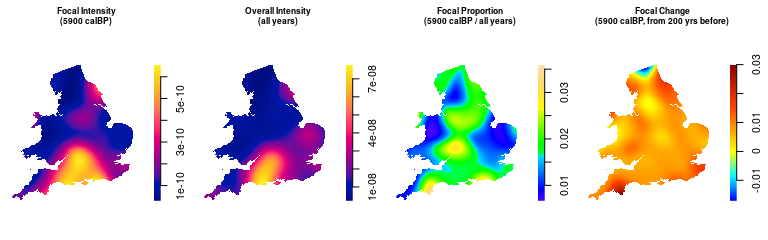### Spatial Permutation Test

When geographic study areas are very large, it becomes inappropriate to assume that there is complete spatial homogeneity in the demographic trajectories of different sub-regions in the study area. At the same time, evaluating such regional divergences is difficult because any increase in spatial scale of a study usually entails also an increase in the heterogeneity of research design and in the overall sampling intensity. rcarbon enables an exploration of spatial heterogeneity in the SPDs that is robust to differences in sampling intensity and provides a permutation-based statistical significance framework (for details of the method see Crema et al. 2017).

In order to carry out a spatial analysis of aggregate radiocarbon dates, we need calibrated dates, bins, and a SpatialPoints class object containing the site locations:

euroevol=subset(euroevol,C14Age<=7200&C14Age>=4200)
eurodates <- calibrate(euroevol$C14Age,euroevol$C14SD,normalised=FALSE,verbose=FALSE)
eurobins <- binPrep(sites=euroevol$SiteID,ages=euroevol$C14Age,h=200)

# Create a data.frame of site locations extracting spatial coordinates
sites <- unique(data.frame(id=euroevol$SiteID,lat=euroevol$Latitude,lon=euroevol$Longitude)) rownames(sites) <- sites$id
sites <- sites[,-1]

# Convert to a SpatialPoints class object:
sp::coordinates(sites) <- c("lon","lat")
sp::proj4string(sites) <- sp::CRS("+proj=longlat +datum=WGS84")

We then need to generate a distance matrix which we will use to define a spatial weighting scheme using the spweights() function. The example below uses a Gaussian decay function (the default) with a bandwidth size of 100 km:

#Compute distance matrix
d <- sp::spDists(sites,sites,longlat=TRUE)
#Compute spatial weights
w <- spweights(d,h=100)

The core function sptest() compares the observed and the expected geometric growth rates rather than the raw SPD. Thus we need to define the breakpoints of our chronological blocks and the overall time range of our analysis. In this case, we examine a sequence of blocks, each 500 years long.

breaks <- seq(8000,5000,-500) #500 year blocks
timeRange <- c(8000,5000) #set the timerange of analysis in calBP, older date first

The function spd2rc() can be used to calculate and visualise the growth rates for specific sequence of blocks.

eurospd = spd(x = eurodates,bins=eurobins,timeRange = timeRange)
plot(spd2rc(eurospd,breaks = breaks))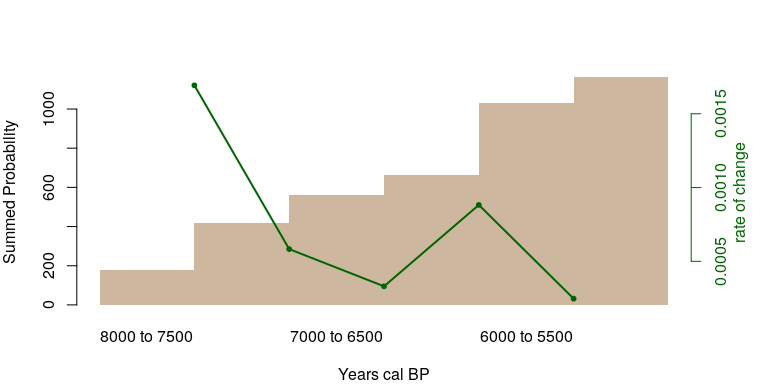In this case, the pan-regional trend shows a positive but declining growth rate through time, except for the transition 6500-6000 to 6000-5500 cal BP when the rate increases slightly.

In order examine whether these dynamics is observed consistently across the study region we conduct a permutation test with the sptest() function:

eurospatial <- sptest(calDates=eurodates, bins=eurobins,timeRange=timeRange, locations=sites,permute="locations",nsim=1000, breaks=breaks, spatialweights=w,ncores=3) 

The output of the function has its own plot() method which provides various ways to display the outcome. The function plots only the point locations, so it is often convenient to load a separate base map. The example below uses the rworldmap package:

library(rworldmap)
base <- getMap(resolution="low") #extract basemap
#extract bounding coordinates of the site distribution
xrange <- bbox(sites)[1,]
yrange <- bbox(sites)[2,]

The plot function requires the definition of an index value (a numerical integer representing the $$i$$-th transition (thus index=1 means first transition, in this case the transition from the time block 8000-7500 to the time block 7500-7000 cal BP), and an option argument, which indicates what needs to be plotted (either the results of the statistical tests or the local estimates of geometric growth rates). The script below examines the transition when the declining growth rate exhibits a short reversion (i.e. 6500-6000 to 6000-5500 cal BP).

## Spatial Permutation Test for Transition 4
par(mar=c(1,1,4,1),mfrow=c(1,2))
plot(base,col="antiquewhite3", border="antiquewhite3",xlim=xrange, ylim=yrange,main="6.5-6 to 6-5.5 kBP \n (Test Results)")
plot(eurospatial,index=4, option="test", add=TRUE, legend=TRUE, legSize=0.7, location="topleft")

## Geometric Growth Rate for Transition 4
plot(base,col="antiquewhite3", border="antiquewhite3", xlim=xrange, ylim=yrange, main="6.5-6 to 6-5.5 kBP \n (Growth Rate)")
plot(eurospatial,index=4, option="raw", add=TRUE, breakRange=c(-0.005,0.005), legend=TRUE,legSize=0.7, location="topleft")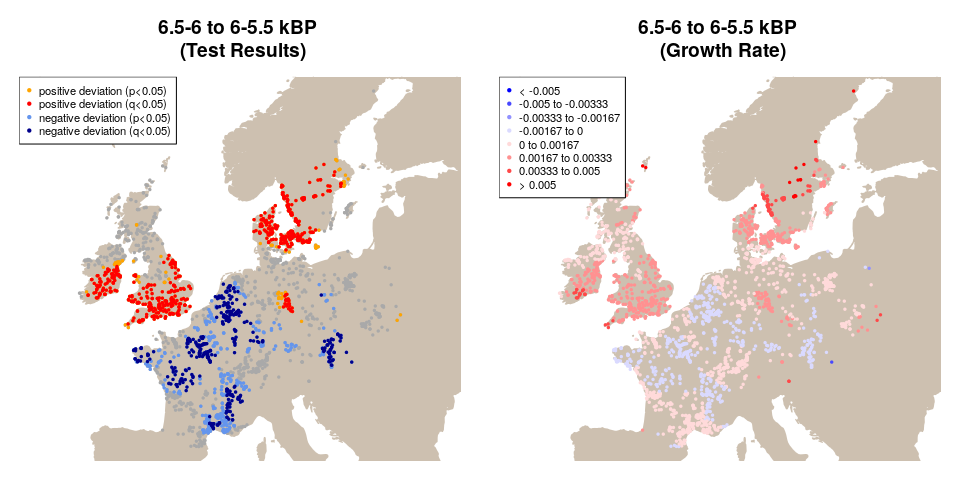The two figures show significant spatial heterogeneity in growth rates. Southern Ireland, Britain, and the Baltic area all exhibit positive growth, while most of France is associated with negative deviations from the pan-regional model. Given the large number of site locations and consequent inflation of type I error, sptest() also calculates a false discovery rate (q-values) using the p.adjust() function with method="fdr". A q-value of 0.05 implies that 5% of the results that have a q-value below 0.05 are false positives.